# Broadcast FM RDS Decode¶

Now to look at some wide FM in the broadcast band, and then decode RDS. Like you do.

RDS is als known as RadioText and RBDS; it's how your car stereo knows the name of the currently playing song when you listen to the radio. This is a really basic implementation of going from raw signal samples to decoding that text.

This involves a bunch of small steps: * Collecting samples at the right tuned frequency and sample rate * Extracting just the FM "audio" signal from that capture * Demoudlating the FM "audio" signal * Filtering out the RDS part of the audio signal * Demodulating/decoding the RDS carrier signal (differential BPSK) to a bitstream * Finding RDS blocks in the bitstream * Unpacking the RDS Blocks in that bitstream

If you're just interested in the boxes and not any of the theory behind them, you should check out gr-rds. This is a GNU Radio Companion implementation of the same thing as you find here. gr-rds is better than this code in every way but one: my goal is learn/teach how this stuff works. I'm eschewing higher-performance implementations because I want this to be transparent.

So, if you're still interested, bear with me as we build a really slow, janky, memory hog of an implementation of an RDS decoder.

(This is part of my series of SDR Snippets, which contains a self-hosted git repo you can clone, 'git clone http://www.joshisanerd.com/projects/sdr_snippets/sdr_snippets.git/'). It's also occasionally sync'd over to github at github/jbm9/sdr_snippets.)

## Let's get started¶

First up, the boilerplate to get our base IPython environment set up to make pretty graphs and whatnot.

In :
%pylab inline

# We'll be doing some signal processing work, so you need scipy
import scipy
import scipy.signal

# Set up our graphs as a bit bigger than the defaults
pylab.rcParams['figure.figsize'] = (14.0, 5.0)

Populating the interactive namespace from numpy and matplotlib



Next, let's specify what we want to capture. We need a center frequency and a sample rate. Here, we're interested in 104.5MHz, and we need at least 100kHz on either side. However, we want to sample "off to the side" to avoid having the DC spike in the middle of our signal. And, looking at the signal, there's some other subcarriers around the station which might be fun to play with, so we'll pull those in as well.

All told, we need roughly 400kHz to capture the station of interest. However, we need that 400kHz "off to one side" of the center, going from 0Hz to +400kHz of our capture. When we capture at 500kHz, we get 500kHz around our center frequency: we get -250kHz to +250kHz. We need 0 to +400kHz, so we need to sample at 800kHz or faster. For reasons that will become clear later, we'll use a bandwidth of 1,140,000 Hz.

You may or may not need an actual gain setting. For some stations here, using gain actually causes problems: the tower is a couple miles from my house. (San Francisco is a pretty small city!)

In :
F_station = int(104.5e6)  # KFOG here in San Francisco

F_offset = 250000         # Offset to capture at, to avoid the DC spike
Fc = F_station - F_offset # Capture center frequency
Fs = int(57e3*20)         # Sample rate
gain = 10                 # Gain
N = int(1024000*8)        # Number of samples to capture, this is ~8 seconds
# The goofy size is to help out RTL-SDR

CAPTURE_METHOD = "rtl"    # "uhd", "rtl", or "file"


### Capture¶

Now, we set up to gather our samples. There are three ways I have available to do this: * From a sample file (downloaded or captured via gqrx/trivial GRC flow/etc) * Capture RTL-SDR dongle * Capture from my Ettus USRP B210

The next bit of code will do whichever of these you ask it to, and put the output data into the numpy array x as a bunch of complex number samples.

In :
# File capture is delightfully simple.  If you have the file, that is.
#
if CAPTURE_METHOD == "file":
x = np.fromfile("broadcast_fm_1140000sps_fc.sdr", dtype="complex64")
psd(x[0:100000], Fs=Fs)
title("Power Spectral Density of data collected via file snarf")

In :
# Capturing from a USRP via UHD is ... complicated
#
# Also, this doesn't free up your UHD device after it's done.
#
if CAPTURE_METHOD == "uhd":
from gnuradio import blocks
from gnuradio import gr
from gnuradio import uhd

# Set up our flow graph
#
tb = gr.top_block()

# Set up the USRP for input
usrp_source = uhd.usrp_source(",", uhd.stream_args(cpu_format="fc32", channels=))
usrp_source.set_samp_rate(Fs)
usrp_source.set_center_freq(Fc, 0)
usrp_source.set_gain(gain, 0)

# Let's try to flush out the first bunch of samples, to let it settle

# Limit ourselves to N samples

# And a sink to dump them into
sink = blocks.vector_sink_c()

tb.connect(usrp_source, skip_head, head, sink) # Can use the handy serial connect method here
tb.run()
tb.stop()
usrp_source.stop()
x = np.array(sink.data()).astype("complex64")

psd(x[0:100000], Fs=Fs)
title("Power Spectral Density of data collected via UHD")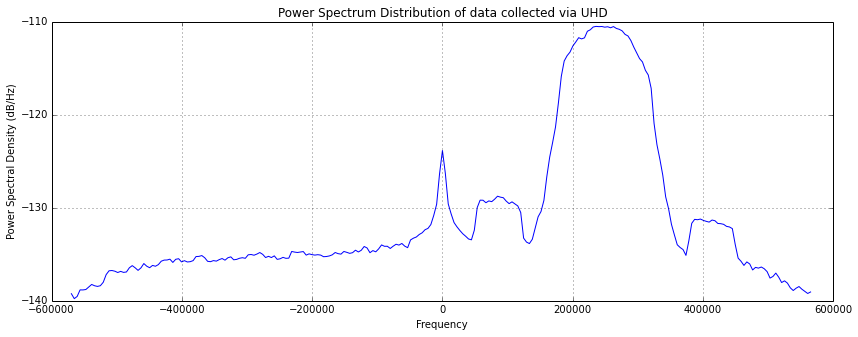In :
if CAPTURE_METHOD == "rtl":
from rtlsdr import RtlSdr

sdr = RtlSdr()

# configure device
sdr.sample_rate = Fs      # Hz
sdr.center_freq = Fc      # Hz
sdr.freq_correction = -52 # PPM
sdr.gain = 0

# If you're getting error code -8 here, that's libusb saying "Overflow."
# It's not an overflow, though, it's actually just that something here wants
# very specially-aligned sample counts.  Try some multiple of 1024000 samples.
# Why is this?  I don't know.
#

sdr.close()
del(sdr)

x = np.array(samples).astype("complex64")
psd(x[0:100000], Fs=Fs)
title("Power Spectral Density of data collected via RTL-SDR")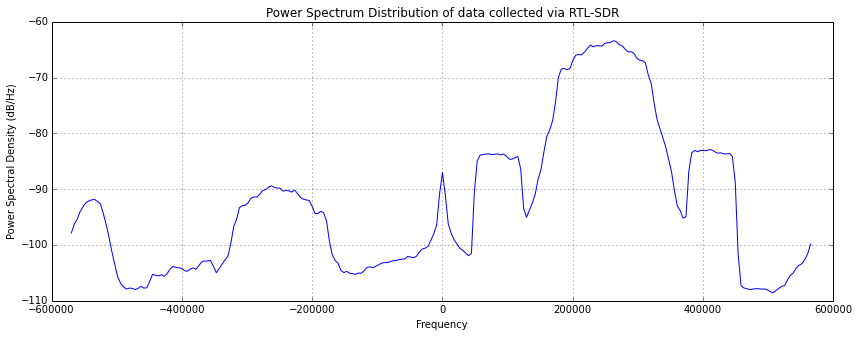After you captured the data above, you should see a "Power Spectral Density" plot. This show you what frequencies are present in your sample file, and how "powerful." This is presented as a diagnostic: you should see a big hump centered at +250,000. Let me make a highlighted version for you. You should see a hump inside the blueish band below

In :
psd(x[0:100000], Fs=Fs)
axvspan(F_offset-100000, F_offset+100000, color="blue", alpha=0.2)
title("PSD of captured signal")
xlabel("Highlighted region should contain FM modulated audio")
"If you don't see a hump in the shaded region below, you may need to choose a different radio station."

Out:
"If you don't see a hump in the shaded region below, you may need to choose a different radio station."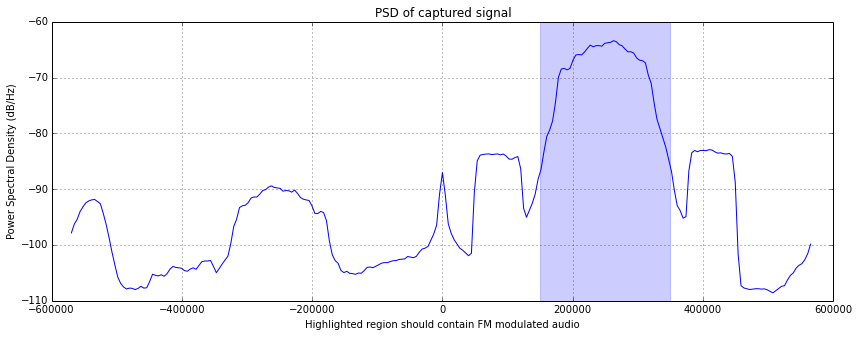## Extracting just the FM signal¶

As you can see in PSD above, there are a bunch of extra little humps all over the place. We don't need all that stuff. Also, we need our signal centered on the FM station to demodulate it. So, we'll create a method for extracting just a single "channel" of signal from the overall capture.

There are three pieces of foundational theory you need here: filtering, mixing, and downsampling. Filtering is just filtering out segments of the frequency contained within a signal. Mixing is how we "move" the center frequency around. Downsampling is how we take our data from one sample rate to a lower sample rate.

Filtering is ... complex (pun intended). The key concept is that you can grab only the frequency ranges you're interested in You can play with the code below to get a feel for it, but a better practical introduction is in "Telecommunication Breakdown". And if you want to understand filters in more depth, I was really happy with Hamming's "Digital Filters". I'll probably also do a bit more of a tutorial on the practical side of really basic filter design. That said, I don't feel like I understand filters well enough yet: there's a lot to them, and really understanding them seems to be very powerful.

Mixing is, for practical intents and purposes, just moving the PSD plot along the x-axis, recentering things as you go. Basically, when you multiply a signal by a sine wave of frequency f, you wind up with a copy of that signal moved up by f Hz (and another one moved down by f Hz, which can be a gotcha). This lets us pull out just our FM segment above.

Finally, we come to downsampling (aka "decimation"). This lets us go from a sample rate of 1MHz to one of 500kHz by just taking every other sample. Or, we could go to 100kHz by taking every 10th sample, etc. The gotcha here is a thing called "aliasing" which has to do with the "Nyquist frequency." Again, "Telecommunication Breakdown" has a good intro to this.

So, here's what we're going to do: * Make a low-pass filter that's the right size for our signal * Mix it up to the center of the band we're after * Apply it to our input signal * Mix our input signal down to be centered at 0Hz * Downsample our signal to the closest whole number divisor of our input sample rate

(This is how it's done within GNU Radio's gr.filter.freq_xlating_fir_filter, if you gave it the taps input from a gr.filter.firdes.low_pass(). You also need to precompute your decimation factor.)

In :
def extract_channel(f_sample, f_center, f_bw, x):
"""Given an input signal x (sampled at f_sample), return a signal centered at f_center with minimal integer-decimated bandwidth > f_bw

Returns Fs_y,y,extract_state: the new sample rate, the new signal, and internal state for your perusal
"""

# Cribbing from the GNURadio xlate, we use their block diagram
#  LPF -> mix up to center -> apply -> downmix -> decimate

# The naive way to do this is to translate the signal down by the
# new center, apply our LPF, and then decimate.
# This has lots of gotachas, and is left as an exercise for the reader.

my_state = {} # Create a cheating backchannel for debug/experimentation

########
# Create a LPF for our target bandwidth
n_taps = 100 # XXX Total random shot in the dark
base_lpf = scipy.signal.remez(n_taps, [0, f_bw, f_bw+(f_sample/2-f_bw)/4, f_sample/2], [1,0], Hz=f_sample)

# Shift this LPF up to our center frequency
#  np.exp(1.0j*fwT0 * np.arange(0, n_taps)) -- This creates a complex sinusoid at Fc Hz
fwT0 = 2.0*np.pi*f_center/f_sample
lpf = base_lpf * np.exp(1.0j*fwT0 * np.arange(0, n_taps))

# Apply the filter
x_filtered = scipy.signal.lfilter(lpf, 1.0, x)

# Mix the filtered data down down by the carrier frequency
x_filtered_downmix = x_filtered * np.exp(-1.0j*fwT0*np.arange(len(x_filtered)))

# Figure out our best decimation rate
dec_rate = int(f_sample / f_bw)

# Actually decimate the data: pull out every dec_rate'th sample
dec_is = np.arange(0, len(x_filtered_downmix), dec_rate)
y = x_filtered_downmix[dec_is]

# Create our cheat output
my_state["n_taps"] = n_taps
my_state["lpf"] = lpf
my_state["base_lpf"] = base_lpf
my_state["fwT0"] = fwT0
my_state["dec_rate"] = dec_rate
# my_state["dec_is"] = dec_is
# my_state["x_filtered"] = x_filtered
# my_state["x_filtered_downmix"] = x_filtered_downmix

return f_sample/dec_rate, y, my_state

In :
Fs_y, y, extract_state = extract_channel(Fs, F_offset, 200000, x)
del(extract_state) # Don't need this right now

psd(y[0:100000], Fs=Fs_y)
title("PSD of the FM modulated audio signal")
"Now, all our signal is between +/- 100kHz, and at sample rate %d" % Fs_y

Out:
'Now, all our signal is between +/- 100kHz, and at sample rate 228000'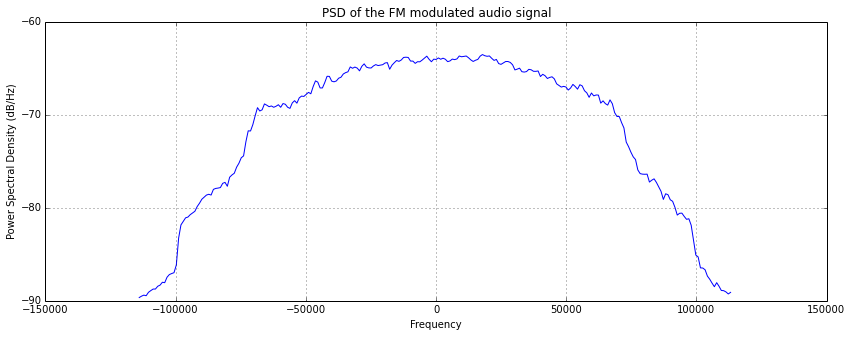At this point, we've pulled out just the FM signal. We've also shrunk the size of our data by roughly 5x, which means everything we do will be roughly 5x faster, which is handy. If you're memory-starved, you might want to del(x) here and then gc() to free up all the RAM our raw signal is hogging up.

Now, to demodulate the FM signal.

## Demodulating the FM Audio¶

We're down to the minimal signal we need, so now let's demodulate the audio out of it.

Let's make the simplifying assumption that we're not pushing our FM modulator too hard, so the frequency shift will never be too big. Then, the effect of moving the frequency around is basically the same as just moving the phase around: as the frequency goes up, the next zero crossing comes sooner, which is like the phase moving forward. Similarly, when the frequency goes down, it's a lot like a phase shift to the right.

So, we're going to use a quadrature demodulator for this. It's delightfully simple/clever.

In :
def decode_quad(x, gain):
# This is tricky to explain, but: we're getting the
# first difference of the phase of our complex samples.
#
# One way to do that would be to take the arctan
# of all the points, then do the diff.
#
# This has a gotcha, though: it's possible to get deltas
# that go the "long way" around the angle circle.  If
# this happens, the output signal is mangled, so we must
# follow our difference with a fixup of the form
#   dtheta[dtheta > np.pi] -= np.pi
#   dtheta[dtheta < np.pi] += np.pi
#
#
# Instead, we lean on the fact that the multiplication
# of two algebraic complex numbers
#     (a+bi)*(c+di) = (ugly expansion via FOIL)
# is the same as multiplying two polar complex numbers:
#     (r0@t0)*(r1@t1) = (r0*r1)@(t0+t1)
# where the radii multiply and the angles add.
#
# Now, the conjugate of a complex number is
#    (a+bi)* = (a-bi).  But in polar form, that's
#    (r@t)* = r@-t
#
# So, to get the difference in angle between two complex
# numbers, we can do
#   arctan(    c0   * conj(c1)   )
#   arctan( (r0@t0) * conj(r1@t1))
#   arctan( (r0@t0) *  (r1@-t1)  )
#   arctan( (r0*r1)@(t0-t1) )
# Now, r0*r1 more or less only matters for sign, so we get
#   sign(r0*r1) * arctan(t0-t1)
#
# So, if we just do this conjugate multiplication all the
# way down, then do arctan, we get our phase changes.
#
# Note that this signal will always be within [-pi,pi], so
# we don't need to do any fixup.
#
xp = x[1:] * np.conj(x[:-1])
retval = gain * np.arctan2(np.imag(xp), np.real(xp))
return retval


Okay, with all that out of the way, let's actually do the demodulation. We need some gain to make it a bit more audible. The right way to do this is involves adjusting for the power loss in the demodulation, but we'll just fudge that for now.

In :
w = decode_quad(y, 10.0)

In :
psd(w, Fs=Fs_y)
title("PSD of our 'audio' signal")
xlabel("Audio is <15kHz (red), pilot tone at 19kHz (green), stereo signal at 38kHz (orange), and DRS at 57kHz (blue)")
axvspan(0,             15000,         color="red", alpha=0.2)
axvspan(19000-500,     19000+500,     color="green", alpha=0.4)
axvspan(19000*2-15000, 19000*2+15000, color="orange", alpha=0.2)
axvspan(19000*3-1500,  19000*3+1500,  color="blue", alpha=0.2)
xlim(0, Fs_y/2)
None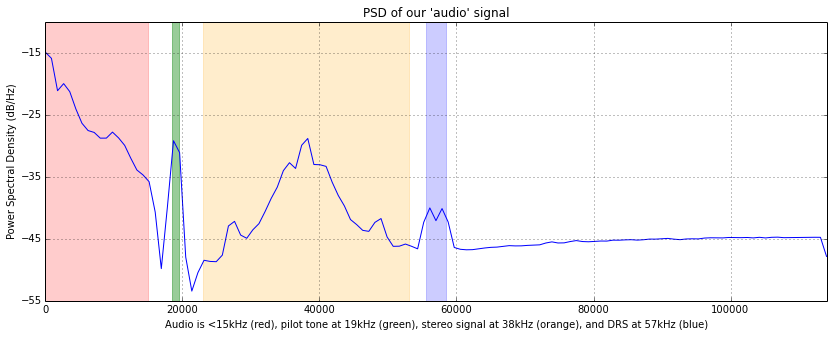Let's put the actual audio out to a file and see if it's actually working, shall we? Note that getting it to a "proper" audio frequency requires interpolating resampling, which I don't want to do. So, I'm writing out an arbitrary bitrate file, then using "aplay" on the command line to play it. This works on Linux. On other systems, you'll have to sort it out for yourself, sorry. Audacity can probably deal with these, which is probably not helpful to say, but I'm going to say it anyway.

In :
dec_audio = int(Fs_y/44100.0)
Fs_audio = Fs_y / dec_audio

# We want to filter out the high-frequency cruft/noise.
# This is roughly "deemphasis" in the way most people talk about FM
lpf_audio = scipy.signal.remez(137, [0,15000, 16000, Fs_y/2], [1,0], Hz=Fs_y)

w_audio = scipy.signal.lfilter(lpf_audio, 1.0, w)
w_audio = w_audio[range(0, len(w_audio), dec_audio)]
psd(w_audio, Fs=Fs_y/dec_audio)
psd(w, Fs=Fs_y)
title("PSD of output audio, before and after preemphasis")
xlim(0, Fs_audio/2)
"Audio is ready to write out, at frequency %d" % Fs_audio

Out:
'Audio is ready to write out, at frequency 45600'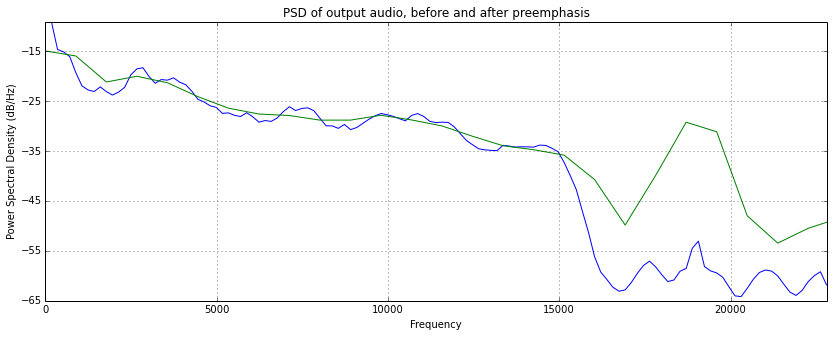In :
scale_factor = 10000 / np.max(np.abs(w_audio))
(w_audio*scale_factor).astype("int16").tofile("wbfm.raw")

In :
from subprocess import call
cli = "aplay -r %d -f S16_LE -t raw -c 1 wbfm.raw" % Fs_audio
call(cli.split(" "))

Out:
0


So, that's us decoding FM radio audio. Pretty neat! Now, let's get to the text in the radio, so we can find out what song we're listening to here.

## Extracting the RDS Text¶

Next up, we need to extract the RDS data. According to the RDS spec, it's effectively binary phase-shift keying (BPSK) with phase deviation of ±90°. The carrier is at 57kHz (3×19kHz), and the symbol rate is 1187.5bps (aka 57kHz/48).

To get at this, we need to filter down to just that 57kHz±1187.5Hz band (with some wiggle room on the edges) signal. We then need to get it down to baseband (centered at zero Hz), and PSK demodulate it.

First, let's do a channel extraction of our data up there. We're not going to use the original channel extraction mechanism, though: our input data is straight floating point, not complex numbers. Instead of turning it into complex numbers, we can save ourselves computation by working with just reals. Also, it's a good opportunity to show each step in the process.

In :
n_taps = 400       # XXX Another totally random shot in the dark
f_center = 57000   # The center frequency we're after

fwT0 = 2.0*np.pi*f_center/Fs_y # The phase velocity of our center

#################
# Remember in the extract_channel(), we talked about the naive way
# being a simple downshift followed by filtering?  We could try that
# here, and also do it with the other method, then compare results.

# Both methods can use this base LPF
base_lpf = scipy.signal.remez(n_taps,
[0, 1250, 2500, Fs_y/2.0],
[1,0],
Hz=Fs_y)

##################
# Here's how we could do this with the BPF.  Note that we need a
# subsequent LPF to clean up the signal afterwards.
#

bpf_57k =  scipy.signal.remez(n_taps,
[0, 55000, 55500, 58500, 59000, Fs_y/2.0],
[0,1,0],
Hz=Fs_y)

# Bandpass filter the input at 57k
v_bpf = scipy.signal.lfilter(bpf_57k, 1.0, w)
# Mix this down to baseband
v_bpf_shifted = v_bpf * np.cos(fwT0 * np.arange(0, len(w)))
# Apply our output filter
u_bpf = scipy.signal.lfilter(base_lpf, 1.0, v_bpf_shifted)

# We can run all the code below to see how it performs.  Then,
# we can try adding or removing the filters above.  As we change
# these, we can measure our system's overall performance, to see
# what does or doesn't work for our current signal.
#
# Here are some results from my signal:
#    # of blocks found, # of packets rx'd, output string
# no 57k bpf, with output filter:
#    222, 96, "THE BAY ____'S ORIGINAL KFOG\r   ________________________________"
# w/ 57k bpf, with output filter:
#    223, 84,  "THE BAY ____'S ORIGI____KFOG____________________________________"]
# w/57k bpf, w/o output filter:
#      62, 0, ['________________________________________________________________']
# no 57k bpf, no output filter:
#    47, 0, ['________________________________________________________________']

# Now, let's try with the shifted-LPF technique, and see how it does:

##################
# Generate our shifted LPF and apply it
#
# Note that we're multiplying by a simple cos here, not a complex
# exponential.  We only need the real cosine component when our
# underlying data isn't complex.
#
lpf_shifted = base_lpf * np.cos(fwT0 * np.arange(0, n_taps))

v_lpf_shift = scipy.signal.lfilter(lpf_shifted, 1.0, w)
u_lpf_shift = v_lpf_shift * np.cos(fwT0 * np.arange(0, len(v_lpf_shift)))
u_lpf_shift = scipy.signal.lfilter(base_lpf, 1.0, u_lpf_shift)

# LPF shift, no output filter:
#    222, 96,  "THE BAY ____'S ORIGINAL KFOG\r   ________________________________"
# LPF shift, with output filter:
#    229, 104, "THE BAY ____'S ORIGINAL KFOG\r   ________________________________"

# It looks like the best performer is the LPF shifted up, followed by LPF of
# the output signal.

# Let's pick one to work with from here on out
u = u_lpf_shift

##################
# Now, to decimate the signal down.
Fsym = 57000/48.0
dec_u = int(Fs_y / (4*Fsym))
Fs_u = Fs_y/dec_u

u = u[range(0, len(u), dec_u)]

#########
# And make some plots of our internal state
psd(w, Fs=Fs_y)
psd(v_bpf_shifted, Fs=Fs_y)
psd(v_lpf_shift  , Fs=Fs_y)
psd(u, Fs=Fs_u)
title("PSD of our input in various states")

figure()
psd(u_bpf[range(0, len(u_bpf), dec_u)], Fs=Fs_u)
psd(u_lpf_shift[range(0, len(u_lpf_shift), dec_u)], Fs=Fs_u)
title("PSD of our output signal")

Fs_u

Out:
4750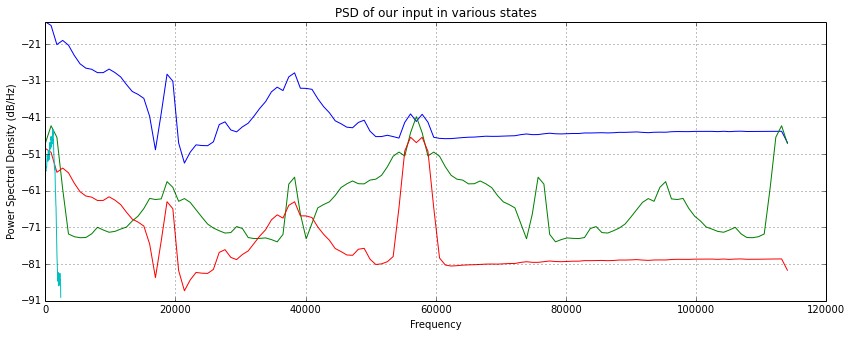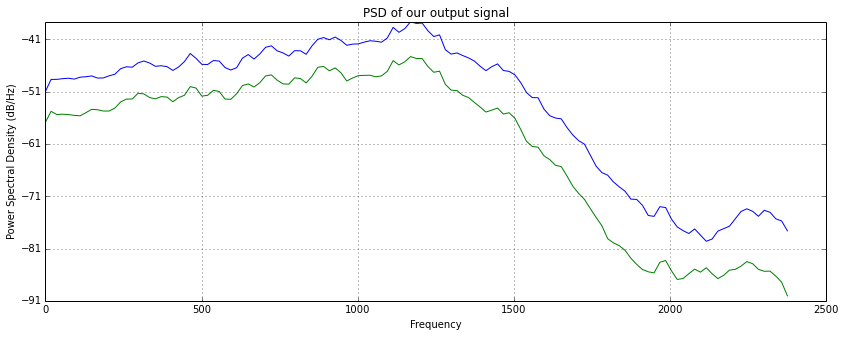Now we're down to just our RDS-bearing signal. We need to do our differential phase-shift-key demodulation/decode. To do this, we need to recover the phase information for our 1187.5Hz carrier signal, then take the difference in phases.

Recovering the phase information from a complex-valued signal is easy: it's a simple matter of arctan. Unfortunately, our input isn't complex: it's just some real floats. We could cons up some complex quadrature signals at our carrier frequency easily enough: just multiply it by a cosine and a sine. The cos term becomes the real part, and the sin term is the imaginary. Boom, instant quadrature. We can then use arctan to recover the phase.

Note that this is BPSK: binary phase shift keying. So, there are two possible values for our phase: zero and not-zero. So, we can just demodulate, then look to see if the absolute value of our phase is more than pi/2.

We also need to decimate this down to just Fsym. Note that Fs_u should be an even multiple of Fsym, so this is still trivial choose-1-of-N downsampling.

In :
dec_sym = int(Fs_u/Fsym)
fwT0 = 2 * np.pi * 2*Fsym / Fs_u

u_i = u * np.cos(np.arange(len(u)) * fwT0)
u_q = u * np.sin(np.arange(len(u)) * fwT0)

u_complex = u_i + 1.0j*u_q

phases = np.arctan2(u_q, u_i)
bitstream_complex = np.abs(phases) <  np.pi/2
bitstream_complex = bitstream_complex[range(0, len(bitstream_complex), dec_sym)]
plot(bitstream_complex[0:100])

title("Bitstream, recovered via creating an I/Q signal, then decode_quad()")
ylim(-0.1, 1.1)

Out:
(-0.1, 1.1)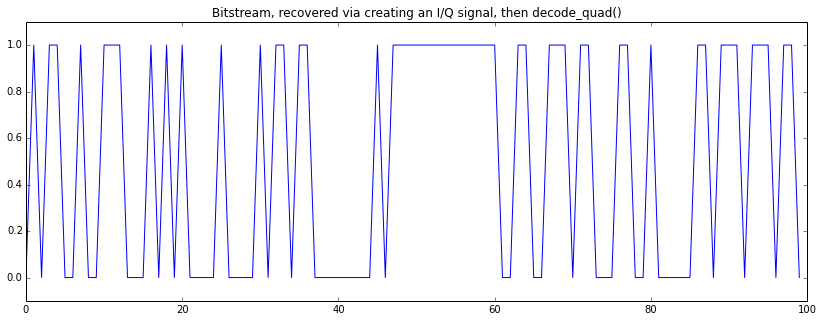However, there is an easier way to do this. Note that we're taking the arctan(kcos(theta), ksin(theta)): this is going to simply be a vector pointing up and to the right or down and to the left, depending on the sign of k. Go pick a bunch of random k and theta values, and draw some diagrams. So, the phase here is either +theta or 180-theta, depending on the sign of k. We don't need to do anything but mix our signal down to baseband and look at the sign of the real value that comes out!

In :
u_at_baseband = u * np.cos(np.arange(len(u)) * fwT0)

bitstream_downmix = (u_i > 0)
bitstream_downmix = bitstream_downmix[[range(0, len(bitstream_downmix), dec_sym)]]
plot(bitstream_downmix[0:100])
title("Bitstream, recovered via downmix")
ylim(-0.1, 1.1)

Out:
(-0.1, 1.1)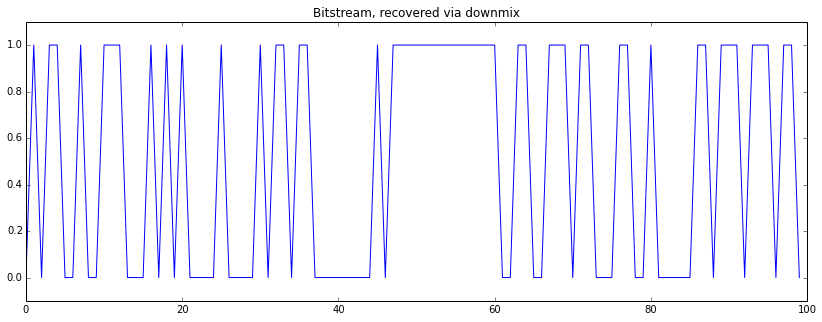And, to "prove" it to ourselves, here's the two signals overlaid for a while:

In :
plot(bitstream_downmix[100:200])
plot(bitstream_complex[100:200])
ylim(-0.1, 1.1)

Out:
(-0.1, 1.1)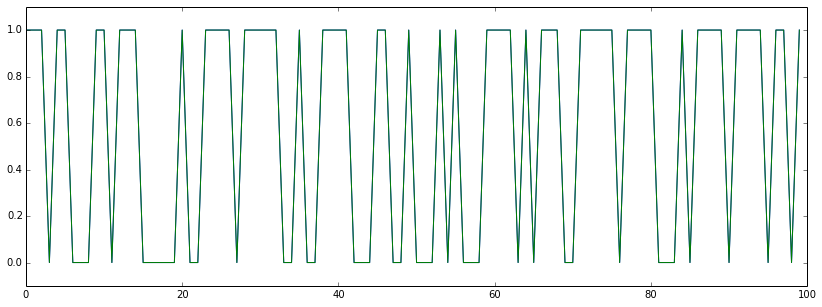Now, we have the signal demodulated, but we still need to remove the differential coding. This part is pretty easy: if there's a 1 at time t, the signal will swap between t and t+1. If there's a zero, it stays the same. So all we need to do is xor the signal against itself, one step off. Alternately, we can just use the != operator, which is what we do here.

In :
bitstream = bitstream_downmix

bitdec = bitstream[1:] != bitstream[:-1]
plot(bitdec[0:100])
ylim(-0.1, 1.1)
title("The differentially-decoded bitstream")
None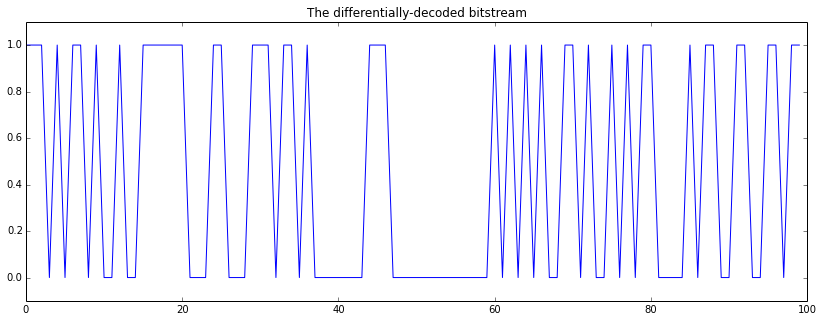### RDS Frame Recovery¶

Now comes the totally-software part: we need to recover the RDS frames from the decoded bitstream. They've thoughtfully made it really easy to find synchronization in their format, which we take full advantage of below.

Each RDS packet is made up of four "blocks." Each block is 26 bits long, with 16 bits of data and a 10 bit checksum. So, each packet is 104 bits long.

The blocks come in five flavors: A,B,C,D, and, probably because it was designed by committee, C'. Each packet contains exactly four blocks, and they're always going to be ABCD or ABC'D. Committees.

Each block checksums to a different "syndrome", so all we need to do to find synchronization is to scan through our whole bitstream, grabbing 26 bits, computing the "syndrome" for that block of bits, and seeing if it matches one of the magic values for A/B/C/D/C'. If it does, we make a note of it. If we get a sequence of four of these in a row (ABCD), we have found a good packet with an absurdly high probability.

Once we find the packets, we can start to take them apart and work with them.

First, we need to get the syndrome checking code going. This is pretty gnarly, and I'm going to skip explaining it for now. Suffice it to say, there's a nice polynomial behind the scenes for the checksum, and blocks have their checksum generated to trigger the appropriate magic value on their output.

In :
import math

# Yoinked from gr-rds, modified to fit our current data better
#
# an implementation of RDS's syndrome calculation
def rds_syndrome(message, m_offset, mlen):
POLY = 0x5B9 # 10110111001, g(x)=x^10+x^8+x^7+x^5+x^4+x^3+1
PLEN = 10
SYNDROME=[383, 14, 303, 663, 748]
OFFSET_NAME=['A', 'B', 'C', 'D', 'C\'']
reg = 0

if((mlen!=16)and(mlen!=26)):
raise ValueError, "mlen must be either 16 or 26"
# start calculation
for i in range(mlen):
reg=(reg<<1)|(message[m_offset+i])
if(reg&(1<<PLEN)):
reg=reg^POLY
for i in range(PLEN,0,-1):
reg=reg<<1
if(reg&(1<<PLEN)):
reg=reg^POLY
checkword=reg&((1<<PLEN)-1)
# end calculation
for i in range(0,5):
if(checkword==SYNDROME[i]):
#print "checkword matches syndrome for offset", OFFSET_NAME[i]
return OFFSET_NAME[i]

return None


Next, we use that syndrome identifying code to scan through our bitstream, finding all candidate blocks. We make note of their offset and their block type. This isn't particularly efficient, but it's a good first step in figuring out what's going on.

In :
my_hits = []

for i in range(len(my_dec)-26):
h = rds_syndrome(my_dec, i, 26)
if h:
my_hits.append( (i, h) )

len(my_hits), my_hits[0:10]

Out:
(210,
[(10, 'B'),
(109, 'B'),
(198, "C'"),
(237, 'D'),
(260, 'A'),
(312, 'D'),
(364, 'B'),
(390, 'C'),
(409, 'B'),
(416, 'D')])


Now we're looking for places where the blocks are aligned with each other on 26 bit boundaries. Let's plot the offset of each block modulo 26 and see what we can see.

In :
plot([r % 26 for r in my_hits])
ylim(-1, 27)
title("26 bit block alignment of each candidate block")
None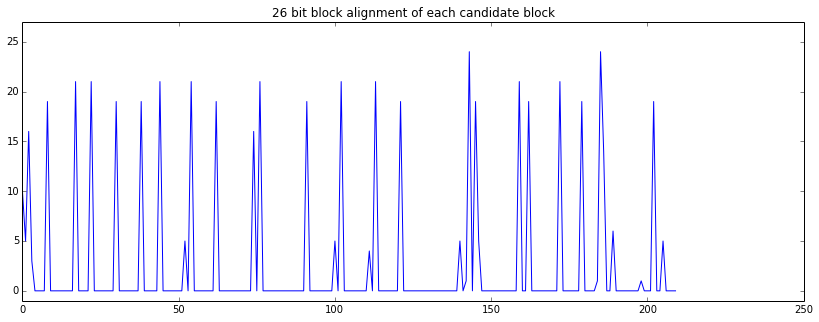In my current view, that looks really great: everything is lined up at 0. This is great, because we can go through and find blocks of ABCD and start pulling them together! (Apologies if this isn't true in the version you're seeing. This is generated from live data every so often...)

### Rough-and-ready RDS Decoder¶

This is a super bare-bones RDS block decoder. It doesn't do much, and, what little it does do, it's probably doing not-quite-correctly. Apologies for that, but it's good enough for my proof-of-concept purposes here.

First, we create a helper method to put together k-bit values from the bitstream, because we're going to do a lot of that.

Then, we create little parse subroutines for each kind of RDS block. We're going to turn these into very rough per-block "structs" to work with in the state machines that accumulate the text.

In :
def _collect_bits(bitstream, offset, n):
"""Helper method to collect a string of n bits, MSB, into an int"""
retval = 0
for i in range(n):
retval = retval*2 + bitstream[offset+i]
return retval

def decode_A(bitstream, offset):
"""Trivial RDS block A decoder"""
return _collect_bits(bitstream, offset, 16)

def decode_B(bitstream, offset):
"""Trivial RDS block B decoder"""
retval = {}

retval["group_type"] = _collect_bits(bitstream, offset, 4)
retval["version_AB"] = "B" if bitstream[offset+4] else "A"
retval["traffic_prog_code"] = bitstream[offset+5]
retval["prog_type"] = _collect_bits(bitstream, offset+6,5)

if retval["group_type"] == 2:
retval["text_segment"] = _collect_bits(bitstream, offset+12, 4)
elif retval["group_type"] == 0:
retval["pi_segment"] = _collect_bits(bitstream, offset+14, 2)

return retval

def decode_C(bitstream, offset):
"""Trivial RDS block C decoder"""
c0 = _collect_bits(bitstream, offset, 8)
c1 = _collect_bits(bitstream, offset+8, 8)

return ( chr(c0), chr(c1))

def decode_Cp(bitstream, offset):
"""Stub RDS block C decoder"""
return None

def decode_D(bitstream, offset):
"""Trivial RDS block D decoder"""
return decode_C(bitstream, offset)

# A lookup table to make it easier to dispatch to subroutines in the code below
decoders = { "A": decode_A, "B": decode_B, "C": decode_C, "C'": decode_Cp, "D": decode_D }

def decode_one(bitstream, offset):
s = rds_syndrome(bitstream, offset, 26)
if None == s:
return None

return (s, decoders[s](bitstream, offset))

In :
hit_parses = []
for i in range(len(my_hits)-3):
if my_hits[i] == "A":
bogus = False
for j,sp in enumerate("ABCD"):
if 26*j != my_hits[i+j] - my_hits[i]:
bogus = True
if my_hits[i+j] != sp:
bogus = True

if not bogus:
for j in range(4):
hit_parses.append( (my_hits[i+j], decode_one(my_dec, my_hits[i+j])))

len(hit_parses), hit_parses[0:10]

Out:
(84,
[(546, ('A', 7846)),
(572,
('B',
{'group_type': 0,
'pi_segment': 2,
'prog_type': 5,
'traffic_prog_code': False,
'version_AB': 'A'})),
(598, ('C', ('\xaa', '\xcd'))),
(624, ('D', ('K', 'I'))),
(650, ('A', 7846)),
(676,
('B',
{'group_type': 0,
'pi_segment': 3,
'prog_type': 5,
'traffic_prog_code': False,
'version_AB': 'A'})),
(702, ('C', ('\xe2', 'f'))),
(728, ('D', ('N', 'G'))),
(754, ('A', 7846)),
(780,
('B',
{'group_type': 2,
'prog_type': 5,
'text_segment': 1,
'traffic_prog_code': False,
'version_AB': 'A'}))])

In :
def accumulate_radiotext(parses):
"""A state machine that accumulates the radio text messages

This takes in a whole list of packet-qualifying blocks (ie:
a list of blocks, but which have been filtered down such that
they always come in ABCD or ABC'D order, and each quad is
adjacent in the bitstream).

Returns a list of states of the radio text as it progresses
through consuming the input packets.
"""
cur_state = ["_"] * 64

retval = [ "".join(cur_state) ]

cursor = None
for blkid, decode in parses:
if blkid == "B":
if decode['group_type'] == 2:
cursor = decode['text_segment'] * 4
else:
cursor = None

if None != cursor:
if blkid == "C":
cur_state[cursor] = decode
cur_state[cursor+1] = decode
retval.append("".join(cur_state))
elif blkid == "D":
cur_state[cursor+2] = decode
cur_state[cursor+3] = decode
retval.append("".join(cur_state))

if blkid == "A" or blkid == "D":
cursor = None

return retval

accumulate_radiotext([ b for (a,b) in hit_parses])


Out:
['________________________________________________________________',
'____BA__________________________________________________________',
'____BAY ________________________________________________________',
"____BAY ____'S__________________________________________________",
"____BAY ____'S O________________________________________________",
"____BAY ____'S O____NA__________________________________________",
"____BAY ____'S O____NAL ________________________________________",
"____BAY ____'S O____NAL ____\r __________________________________",
"____BAY ____'S O____NAL ____\r   ________________________________",
"TH__BAY ____'S O____NAL ____\r   ________________________________",
"THE BAY ____'S O____NAL ____\r   ________________________________",
"THE BAY ____'S ORI__NAL ____\r   ________________________________",
"THE BAY ____'S ORIGINAL ____\r   ________________________________",
"THE BAY ____'S ORIGINAL KF__\r   ________________________________",
"THE BAY ____'S ORIGINAL KFOG\r   ________________________________"]

In :
def accumulate_b0text(parses):
cur_state = ["_"] * 8

retval = [ "".join(cur_state) ]

cursor = None
for blkid, decode in parses:
if blkid == "B":
if decode['group_type'] == 0:
cursor = decode['pi_segment'] * 2
else:
cursor = None

if None != cursor:
if blkid == "D":
cur_state[cursor] = decode
cur_state[cursor+1] = decode
retval.append("".join(cur_state))
if blkid == "A" or blkid == "D":
cursor = None

return retval
accumulate_b0text([ b for (a,b) in hit_parses])

Out:
['________',
'____KI__',
'____KING',
'BR__KING',
'BR__KING',
'WA__KING',
'WA__  NG',
'WA__    ',
'WA__    ',
'WA__    ',
'WA__    ',
'WA__    ',
'WA__    ',
'WA__    ',
'WA__    ']

In :
from collections import defaultdict

n_grouptype = defaultdict(int)

for o, d in hit_parses:
if d == "B":
n_grouptype[d['group_type']] += 1

n_grouptype

Out:
defaultdict(<type 'int'>, {0: 14, 2: 7})

In :